Problem

# Calculating the space time for parallel reactions. m-Xylene is reacted over a ZSM-5 zeolit...

Calculating the space time for parallel reactions. m-Xylene is reacted over a ZSM-5 zeolite catalyst. The following parallel elementary reactions were found to occur in excess H2 [Ind. Eng. Chem. Res., 27, 942 (1988)] :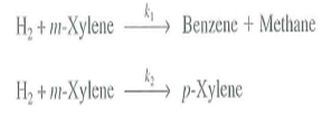(a) Calculate the PFR volume to achieve 85% conversion of m-xylene in packed-bed reactor. Plot the overall selectivity and yields as a function of τ. The specific reaction rates are k1 = 0.22 s-1 and k2 = 0.71 s-1 at 673°C.

A mixture of 75% m-xylene and 25% inerts is fed to a tubular reactor at a volumetric flow rate of 200 dm3/s and a total concentration of 0.05 mol/clm3 As a first approximation, neglect any other reactions such as

the reverse reactions and isomerization to o-xylene.

(b) Suppose that E1 = 20,000 cal/mol and E2 = 10,000 cal/mol, what temperature would you recommend to maximize the formation of p-xylene in a 2000-clm3 CSTR

#### Step-by-Step Solution

Solution 1

(a) The following parallel elementary reactions occur in excess of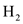.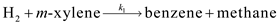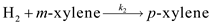As the reactions occur in excess of, the rate of the each reaction depends on the concentration of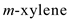. Rewrite the equivalent reactions as follows: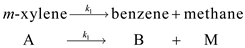And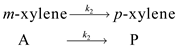The following information is available for the above reactions occurring in PBR.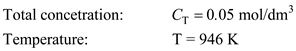A mixture of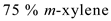and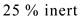was fed to the PBR. Calculate the PFR volume:

Write the rate laws as follows:The rate laws in terms of molar flow rate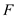per volume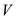are written as follows:The mole balance can be written as follows: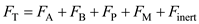Solve the differential equations using Polymath as follows: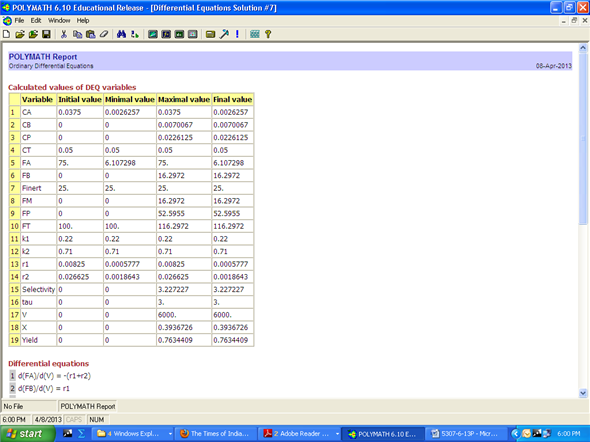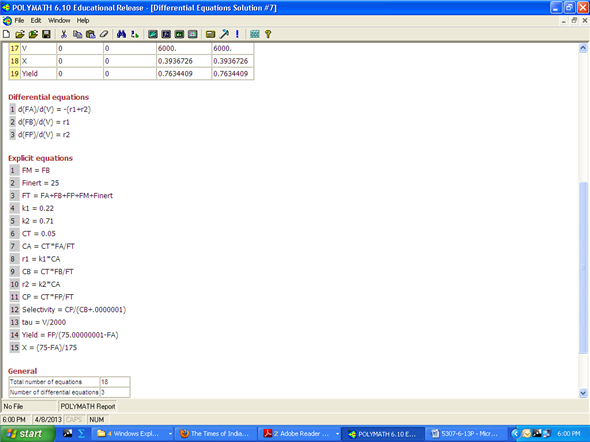The plot of conversion, overall selectivity and overall yield as a function of space time is as follows: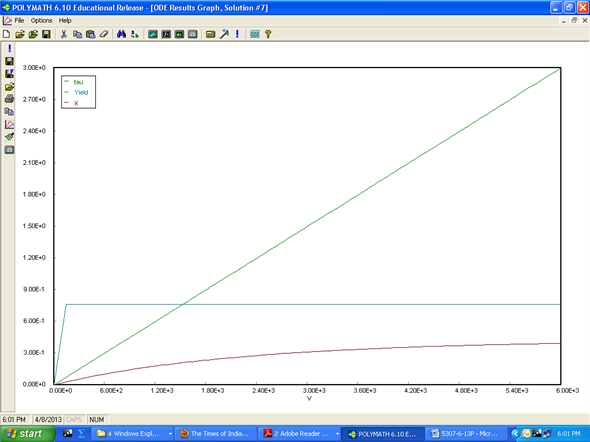Hence, the PFR volume to achieve 85% conversion is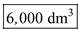.

(b) If the activation energies are;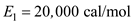,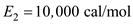and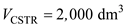. Calculate the temperature to maximize the formation of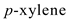.

Write the rate laws as follows:Integrate the rate equations:The Arrhenius equation is as follows:Here,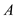is the frequency factor and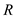is the gas constant.

The mole balance for CSTR is written as follows:Solve the integrated forms of the rate laws as follows: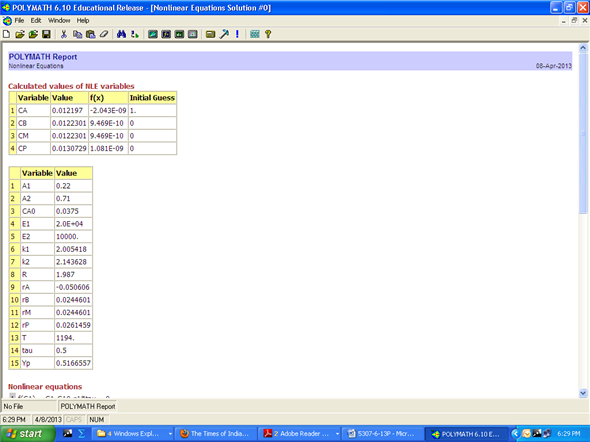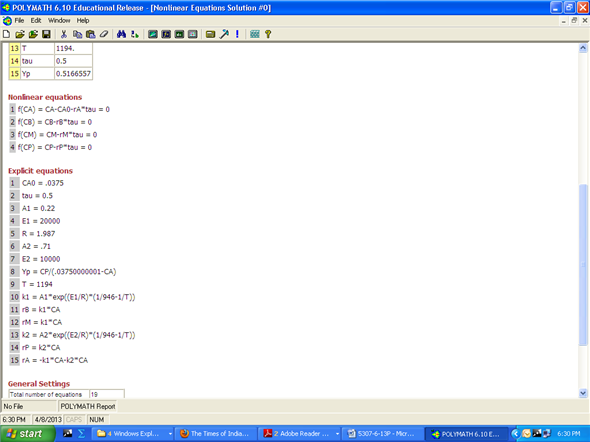Hence, the temperature is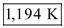and the concentration of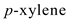is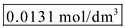.